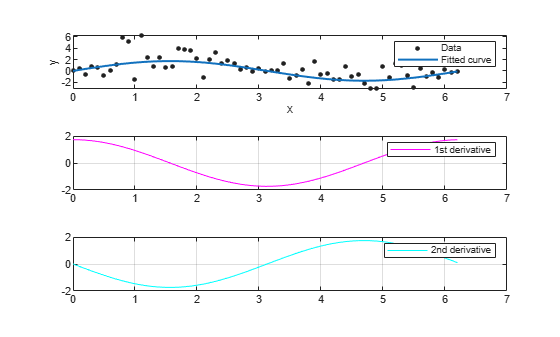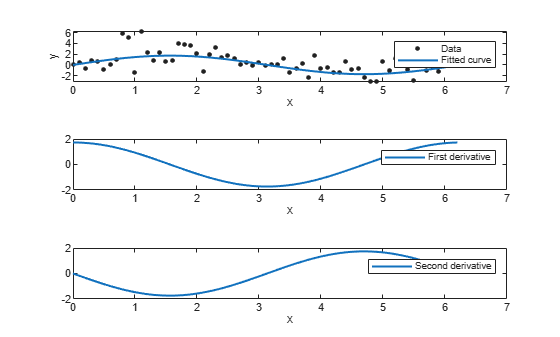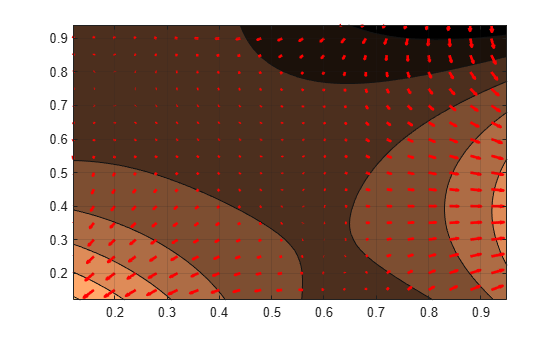Main Content

# differentiate

Differentiate `cfit` or `sfit` object

## Syntax

``fx = differentiate(FO, X)``
``[fx, fxx] = differentiate(FO, X)``
``[fx, fy] = differentiate(FO, X, Y)``
``[fx, fy] = differentiate(FO, [X, Y])``
``[fx, fy, fxx, fxy, fyy] = differentiate(FO, ...)``

## Description

``` NoteUse these syntaxes for `cfit` objects. `fx = differentiate(FO, X)` differentiates the `cfit` object `FO` at the points specified by the vector `X` and returns the result in `fx`.```

example

````[fx, fxx] = differentiate(FO, X)` differentiates the `cfit` object `FO` at the points specified by the vector `X` and returns the result in `fx` and the second derivative in `fxx`.```
``` NoteUse these syntaxes for `sfit` objects. `[fx, fy] = differentiate(FO, X, Y)` differentiates the surface `FO` at the points specified by `X` and `Y` and returns the result in `fx` and `fy`.`FO` is a surface fit (`sfit`) object generated by the `fit` function.`X` and `Y` must be double-precision arrays and the same size and shape as each other.All return arguments are the same size and shape as `X` and `Y`. If `FO` represents the surface $z=f\left(x,y\right)$, then `FX` contains the derivatives with respect to x, that is, $\frac{df}{dx}$, and `FY` contains the derivatives with respect to y, that is, $\frac{df}{dy}$. ```
````[fx, fy] = differentiate(FO, [X, Y])`, where `X` and `Y` are column vectors, allows you to specify the evaluation points as a single argument.```
````[fx, fy, fxx, fxy, fyy] = differentiate(FO, ...)` computes the first and second derivatives of the surface fit object `FO`.`fxx` contains the second derivatives with respect to `x`, that is, $\frac{{\partial }^{2}f}{\partial {x}^{2}}$.`fxy` contains the mixed second derivatives, that is, $\frac{{\partial }^{2}f}{\partial x\partial y}$.`fyy` contains the second derivatives with respect to `y`, that is, $\frac{{\partial }^{2}f}{\partial {y}^{2}}$. ```

## Examples

collapse all

Create a baseline sinusoidal signal.

```xdata = (0:.1:2*pi)'; y0 = sin(xdata);```

Add response-dependent Gaussian noise to the signal.

```noise = 2*y0.*randn(size(y0)); ydata = y0 + noise;```

Fit the noisy data with a custom sinusoidal model.

```f = fittype('a*sin(b*x)'); fit1 = fit(xdata,ydata,f,'StartPoint',[1 1]);```

Find the derivatives of the fit at the predictors.

`[d1,d2] = differentiate(fit1,xdata);`

Plot the data, the fit, and the derivatives.

```subplot(3,1,1) plot(fit1,xdata,ydata) % cfit plot method subplot(3,1,2) plot(xdata,d1,'m') % double plot method grid on legend('1st derivative') subplot(3,1,3) plot(xdata,d2,'c') % double plot method grid on legend('2nd derivative')```You can also compute and plot derivatives directly with the `cfit` `plot` method, as follows:

```figure plot(fit1,xdata,ydata,{'fit','deriv1','deriv2'})```The `plot` method, however, does not return data on the derivatives, unlike the `differentiate` method.

You can use the `differentiate` method to compute the gradients of a fit and then use the `quiver` function to plot these gradients as arrows. This example plots the gradients over the top of a contour plot.

Create the derivation points and fit the data.

```x = [0.64;0.95;0.21;0.71;0.24;0.12;0.61;0.45;0.46;... 0.66;0.77;0.35;0.66]; y = [0.42;0.84;0.83;0.26;0.61;0.58;0.54;0.87;0.26;... 0.32;0.12;0.94;0.65]; z = [0.49;0.051;0.27;0.59;0.35;0.41;0.3;0.084;0.6;... 0.58;0.37;0.19;0.19]; fo = fit( [x, y], z, 'poly32', 'normalize', 'on' ); [xx, yy] = meshgrid( 0:0.04:1, 0:0.05:1 );```

Compute the gradients of the fit using the `differentiate` function.

`[fx, fy] = differentiate( fo, xx, yy );`

Use the `quiver` function to plot the gradients.

```plot( fo, 'Style', 'Contour' ); hold on h = quiver( xx, yy, fx, fy, 'r', 'LineWidth', 2 ); hold off colormap( copper )```If you want to use derivatives in an optimization, you can, for example, implement an objective function for `fmincon` as follows.

`function [z, g, H] = objectiveWithHessian( xy )`

` % The input xy represents a single evaluation point`

` z = f( xy );`

` if nargout > 1`

` [fx, fy, fxx, fxy, fyy] = differentiate( f, xy );`

` g = [fx, fy];`

` H = [fxx, fxy; fxy, fyy];`

` end`

` end`

## Input Arguments

collapse all

Function to differentiate, specified as a `cfit` object for curves or as a `sfit` object for surfaces.

Points at which to differentiate the function, specified as a vector. For surfaces, this argument must have the same size and shape of Y.

Points at which to differentiate the function, specified as a vector. For surfaces, this argument must have the same size and shape of X.

## Output Arguments

collapse all

First derivative of the function, returned as a vector of the same size and shape of X and Y.

If FO is a surface, $z=f\left(x,y\right)$, then fx contains the derivatives with respect to `x`.

Second derivative of the function, returned as a vector of the same size and shape of X and Y.

If FO is a surface, $z=f\left(x,y\right)$, then fxx contains the second derivatives with respect to `x`.

First derivative of the function, returned as a vector of the same size and shape of X and Y.

If FO is a surface, $z=f\left(x,y\right)$, then fy contains the derivatives with respect to `y`.

Second derivative of the function, returned as a vector of the same size and shape of X and Y.

If FO is a surface, $z=f\left(x,y\right)$, then fyy contains the second derivatives with respect to `y`.

Mixed second derivative of the function, returned as a vector of the same size and shape of X and Y.

## Tips

For library models with closed forms, the toolbox calculates derivatives analytically. For all other models, the toolbox calculates the first derivative using the centered difference quotient

`$\frac{df}{dx}=\frac{f\left(x+\Delta x\right)-f\left(x-\Delta x\right)}{2\Delta x}$`

where x is the value at which the toolbox calculates the derivative, $\Delta x$ is a small number (on the order of the cube root of `eps`), $f\left(x+\Delta x\right)$ is `fun` evaluated at $x+\Delta x$, and $f\left(x-x\Delta \right)$ is `fun` evaluated at $x-\Delta x$.

The toolbox calculates the second derivative using the expression

`$\frac{{d}^{2}f}{d{x}^{2}}=\frac{f\left(x+\Delta x\right)+f\left(x-\Delta x\right)-2f\left(x\right)}{{\left(\Delta x\right)}^{2}}$`

The toolbox calculates the mixed derivative for surfaces using the expression

`$\frac{{\partial }^{2}f}{\partial x\partial y}\left(x,y\right)=\frac{f\left(x+\Delta x,y+\Delta y\right)-f\left(x-\Delta x,y+\Delta y\right)-f\left(x+\Delta x,y-\Delta y\right)+f\left(x-\Delta x,y-\Delta y\right)}{4\Delta x\Delta y}$`

## See Also

### Topics

Introduced before R2006a

## Support

#### Machine Learning Challenges: Choosing the Best Classification Model and Avoiding Overfitting

Download white paper